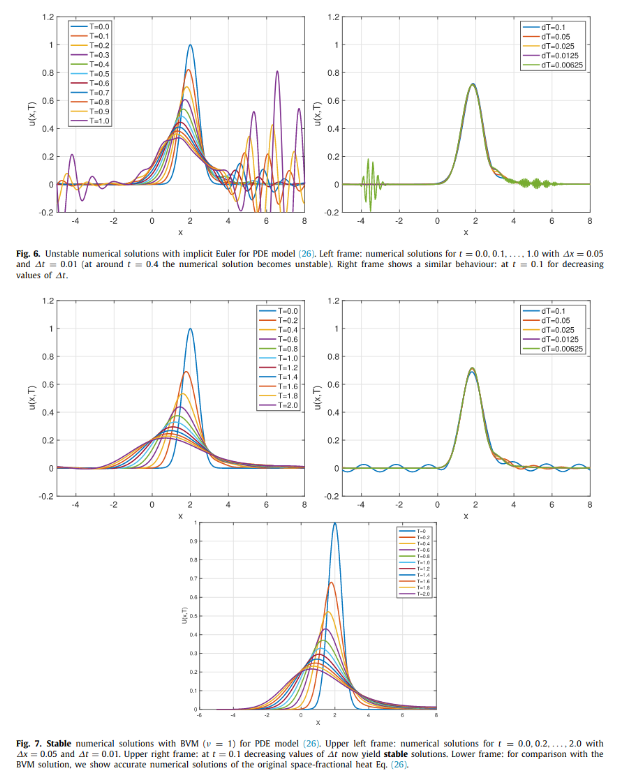# A generalized midpoint-based boundary value method for unstable partial differential equations### Abstract

We present a class of Boundary Value Methods (BVMs) that can be applied to semi-stable and unstable Partial Differential Equation (PDE) models. Step-by-step (initial value) methods, such as Runge–Kutta and linear multistep methods have numerical stability regions which do not intersect with certain regions of the complex plane that are significant to the time-integration of unstable PDEs. BVMs, which need extra numerical conditions at the final time, are global methods and are, in some sense, free of such barriers. We discuss BVMs based on generalized midpoint methods, combined with appropriate numerical initial and final conditions. The stability regions of these methods intersect with a significant part of the complex plane. In several numerical experiments we will illustrate the usefulness of such methods when applied to PDE models, such as a dispersive wave equation, a space-fractional PDE and the backward heat equation.

Type
Publication
Journal of Computational and Applied Mathematics 2023